# Killer Cars for TRS-80

#### By Richard Kipp

This game was originally published in Softside magazine (Vol 5, Number6, March, 1982), for which I was paid the princely sum of \$100.00 US. As this was my first published work, I was thrilled (I'd have let them print it for free). One of the few downsides of SoftSide magazine was its lack of feedback. I never knew whether people liked the game, hated it, or ignored it.

Thanks to James Jacobs, who has been searching for my source code for the last 22 years (I guess he liked it), I dug up my printout of the code and am publishing it on the internet. I sold my TRS-80 about five years ago (for \$5.00 including a lot of game software), so I don't have a 'native' version of the source code, and if I did I don't know how I'd have read a TRS-80 audio data cassette into a modern computer.

Following are scans of Source code, and of the article I sent in with the source code.

Thanks are due to my brother Dan for searching the internet for Killer Cars references, and to my brother Rod for his excellent scanning and photo cleanup work. Thanks!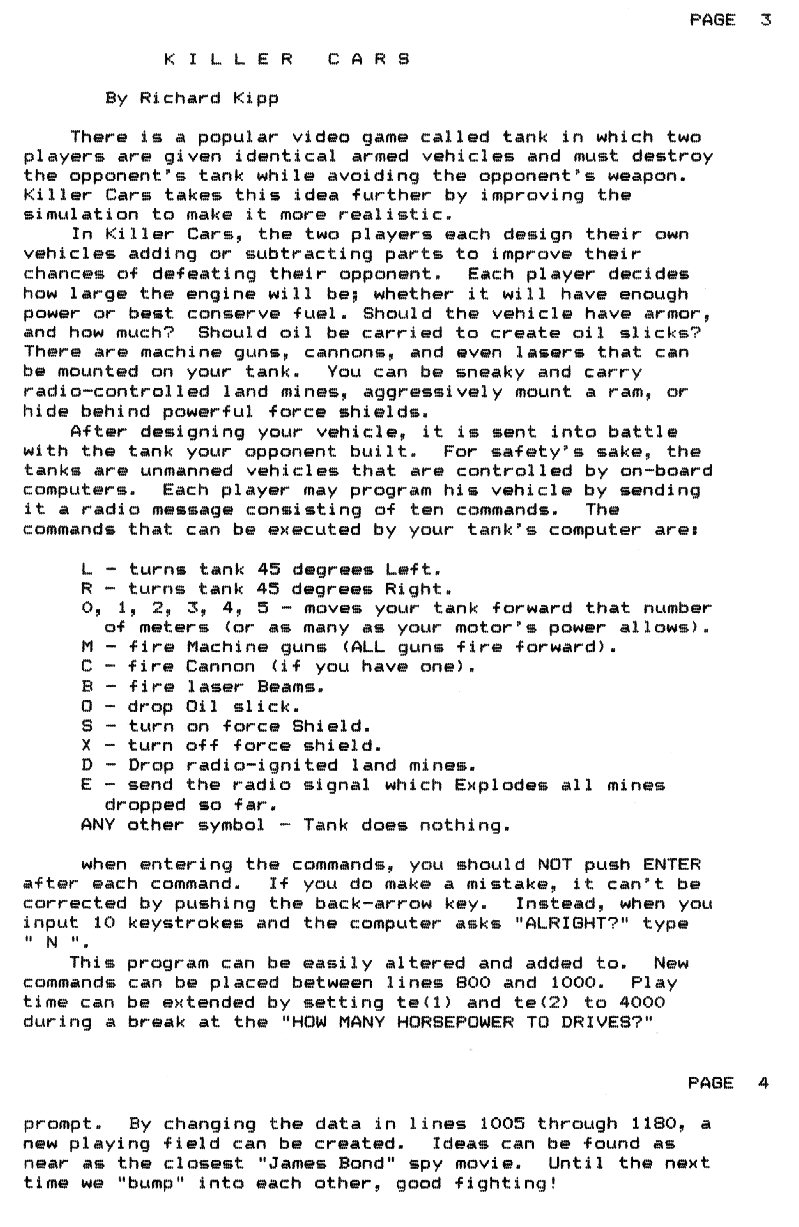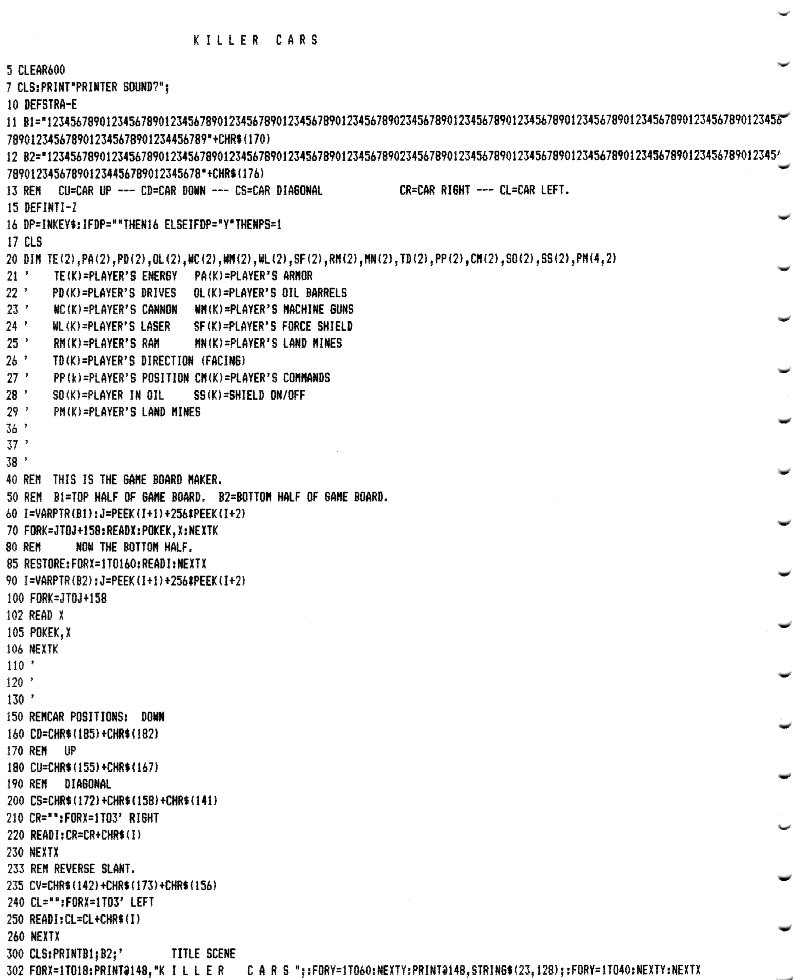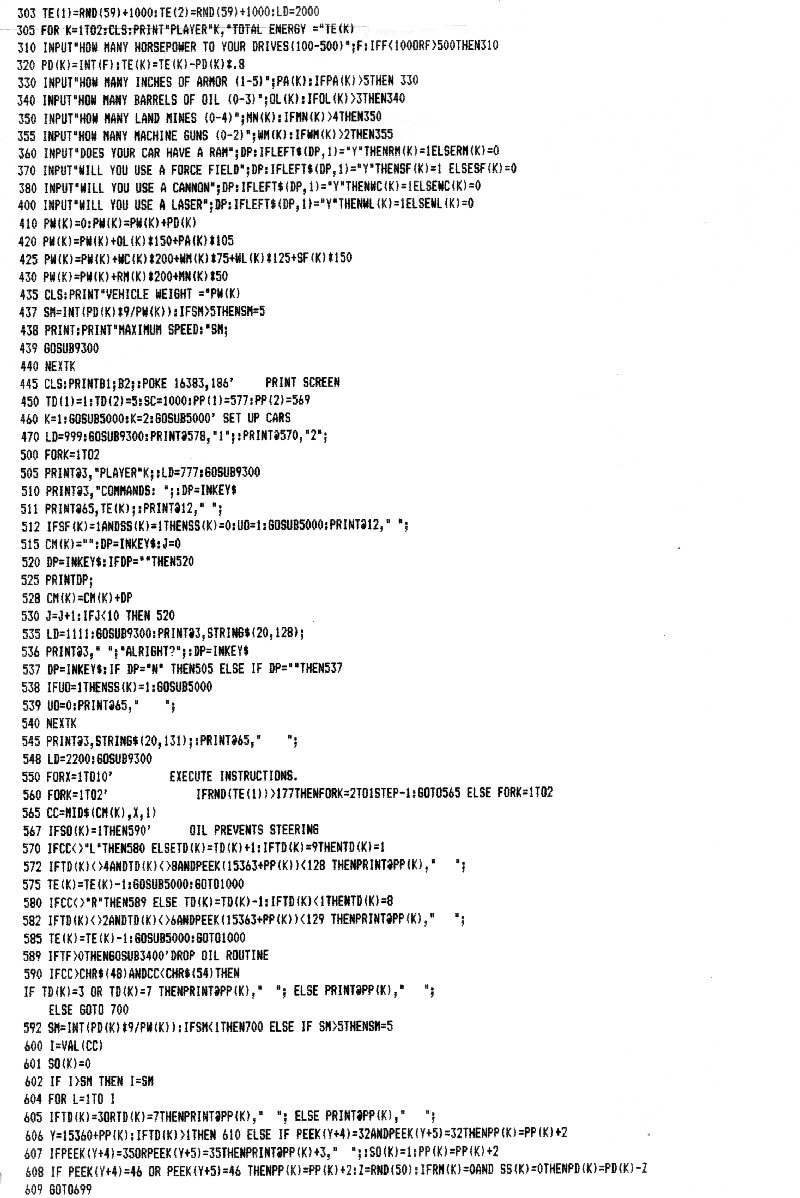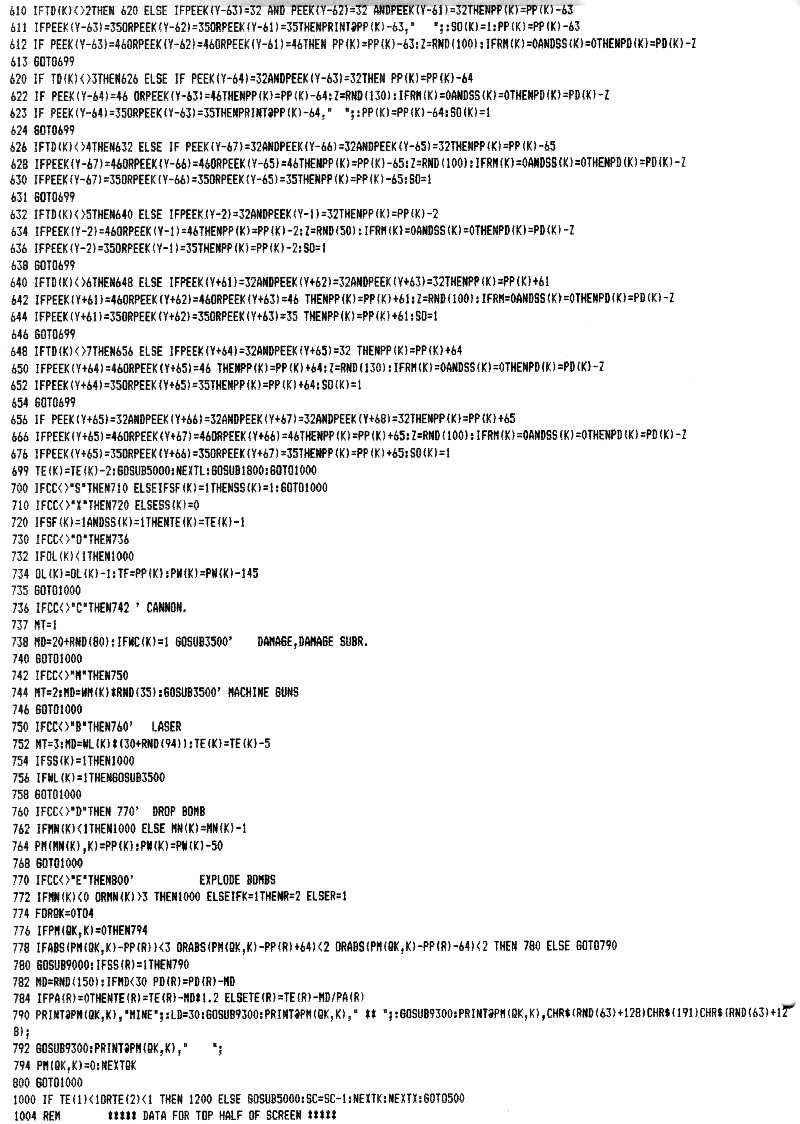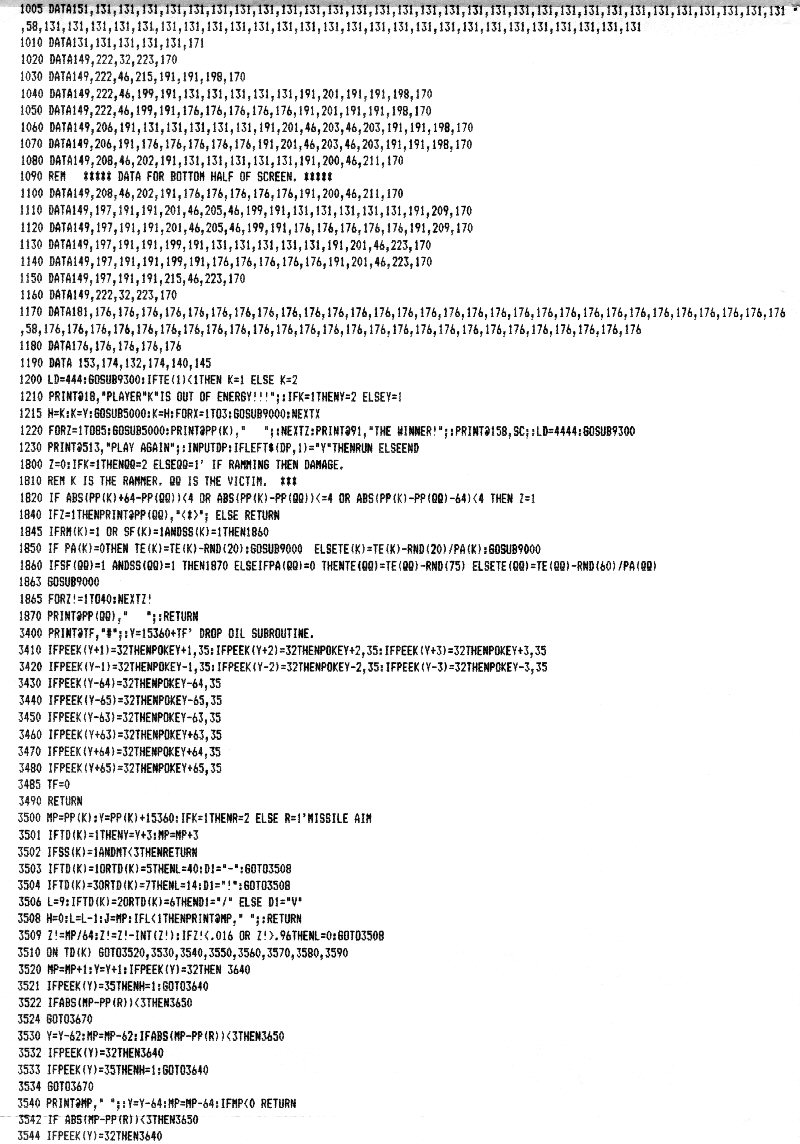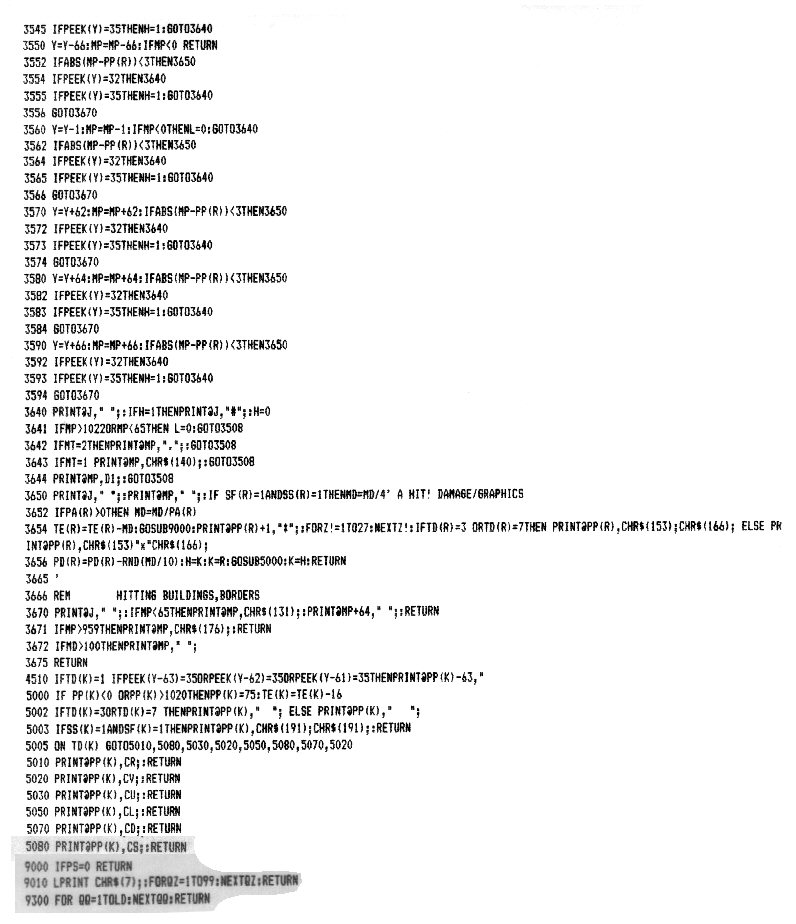Eventually I'll find my copy of that issue of the magazine and scan in the whole article.

UPDATE: 07/12/2005
Here are some erroneous lines that were corrected after publication:
• Line 642:
• as printed:
• IFPEEK(Y+61)=46ORPEEK(Y+62)=46ORPEEK(Y+63)=46 THENPP(K)=PP(K)+61:Z=RND(100):IFRM=0ANDSS(K)=0THENPD(K)=PD(K)-Z
• corrected to:
• IFPEEK(Y+61)=46ORPEEK(Y+62)=46ORPEEK(Y+63)=46 THENPP(K)=PP(K)+61:Z=RND(100):IFRM(K)=0ANDSS(K)=0THENPD(K)=PD(K)-Z
• Line 4510:
• as printed:
• IFTD(K)=1 IFPEEK(Y-63)=35ORPEEK(Y-62)=35ORPEEK(Y-61)=35THENPRINT@PP(K)-63,"
• corrected to:
• IFTD(K)=1 IFPEEK(Y-63)=35ORPEEK(Y-62)=35ORPEEK(Y-61)=35THENPRINT@PP(K)-63," ";